# 4. Feature Engineering¶

It is a process of creating new features or tranforming exisitng features to get most out of the data.

Some examples of creating new features:

• Length of text field.
• % of characters that are punctuation in the text
• % of characters that are capitalized

To create some of the new features, we could apply some of the Transformation techniques such as:

• Power Transformations (square, square root, etc.)
• Standardizing data

### Feature Creation¶

In :
import pandas as pd

data = pd.read_csv('SMSSpamCollection.tsv', sep = '\t', names = ['label', 'body_text'])


Out:
label body_text
0 ham I've been searching for the right words to tha...
1 spam Free entry in 2 a wkly comp to win FA Cup fina...
2 ham Nah I don't think he goes to usf, he lives aro...
3 ham Even my brother is not like to speak with me. ...
4 ham I HAVE A DATE ON SUNDAY WITH WILL!!

#### Creating a text measure length feature¶

We will assume that spam messages tend to be longer than real text messages. We will create this feature and then we will explore whether our hypothesis is accurate.

In :
# to calculate the correct length of messages, we will deduct count of whitespaces
data['body_len'] = data['body_text'].apply(lambda x: len(x) - x.count(" "))

Out:
label body_text body_len
0 ham I've been searching for the right words to tha... 160
1 spam Free entry in 2 a wkly comp to win FA Cup fina... 128
2 ham Nah I don't think he goes to usf, he lives aro... 49
3 ham Even my brother is not like to speak with me. ... 62
4 ham I HAVE A DATE ON SUNDAY WITH WILL!! 28

#### Creating a % of text that is punctuation feature¶

In :
import string

# defining a function
def count_punct(text):
count = sum([1 for char in text if char in string.punctuation]) # total count of punctuation
return round(count/(len(text) - text.count(" ")), 3)*100 # No. of punctuations divided by length of message (excluding whitespaces as we did above)

# applying the above created function
data['punc%'] = data['body_text'].apply(lambda x: count_punct(x))

Out:
label body_text body_len punc%
0 ham I've been searching for the right words to tha... 160 2.5
1 spam Free entry in 2 a wkly comp to win FA Cup fina... 128 4.7
2 ham Nah I don't think he goes to usf, he lives aro... 49 4.1
3 ham Even my brother is not like to speak with me. ... 62 3.2
4 ham I HAVE A DATE ON SUNDAY WITH WILL!! 28 7.1

### Evaluting Feature¶

In :
# importing packages
from matplotlib import pyplot
%matplotlib inline
import numpy as np


#### Buidling 2 Histogram to look at the distribution of body length for spam and ham¶

In :
# definining bins for hitogram using numpy
bins = np.linspace(0, 200, 40)

# plot for spam distribution
pyplot.hist(data[data['label'] == 'spam']['body_len'], bins, alpha=0.5, density=True, label='spam')

# plot for ham distribution
pyplot.hist(data[data['label'] == 'ham']['body_len'], bins, alpha=0.5, density=True, label='ham')

#creating legend
pyplot.legend(loc='upper left')

pyplot.show()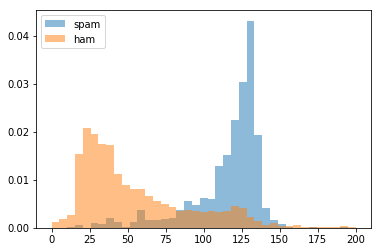#### Buidling 2 Histogram to look at the % of text that is punctuation for spam and ham¶

In :
# definining bins for hitogram using numpy
bins = np.linspace(0, 50, 40) # this is in %

# plot for spam distribution
pyplot.hist(data[data['label'] == 'spam']['punc%'], bins, alpha=0.5, density=True, label='spam')

# plot for ham distribution
pyplot.hist(data[data['label'] == 'ham']['punc%'], bins, alpha=0.5, density=True, label='ham')

#creating legend
pyplot.legend(loc='upper right')

pyplot.show()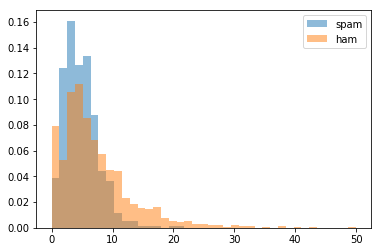### Feature Transformations¶

In :
import pandas as pd

data = pd.read_csv('SMSSpamCollection.tsv', sep = '\t', names = ['label', 'body_text'])


Out:
label body_text
0 ham I've been searching for the right words to tha...
1 spam Free entry in 2 a wkly comp to win FA Cup fina...
2 ham Nah I don't think he goes to usf, he lives aro...
3 ham Even my brother is not like to speak with me. ...
4 ham I HAVE A DATE ON SUNDAY WITH WILL!!

#### Creating a text measure length feature¶

In :
# to calculate the correct length of messages, we will deduct count of whitespaces
data['body_len'] = data['body_text'].apply(lambda x: len(x) - x.count(" "))

Out:
label body_text body_len
0 ham I've been searching for the right words to tha... 160
1 spam Free entry in 2 a wkly comp to win FA Cup fina... 128
2 ham Nah I don't think he goes to usf, he lives aro... 49
3 ham Even my brother is not like to speak with me. ... 62
4 ham I HAVE A DATE ON SUNDAY WITH WILL!! 28

#### Creating a % of text that is punctuation feature¶

In :
import string

# defining a function
def count_punct(text):
count = sum([1 for char in text if char in string.punctuation]) # total count of punctuation
return round(count/(len(text) - text.count(" ")), 3)*100 # No. of punctuations divided by length of message (excluding whitespaces as we did above)

# applying the above created function
data['punc%'] = data['body_text'].apply(lambda x: count_punct(x))

Out:
label body_text body_len punc%
0 ham I've been searching for the right words to tha... 160 2.5
1 spam Free entry in 2 a wkly comp to win FA Cup fina... 128 4.7
2 ham Nah I don't think he goes to usf, he lives aro... 49 4.1
3 ham Even my brother is not like to speak with me. ... 62 3.2
4 ham I HAVE A DATE ON SUNDAY WITH WILL!! 28 7.1
In :
# importing packages
from matplotlib import pyplot
%matplotlib inline
import numpy as np


#### Buidling 2 Histogram to look at the full distribution of body length for spam and ham¶

In :
# definining bins for hitogram using numpy
bins = np.linspace(0, 200, 40)

# plot for spam distribution
pyplot.hist(data['body_len'], bins) # changes done here

# title
pyplot.title('Body Length Distribution')

pyplot.show()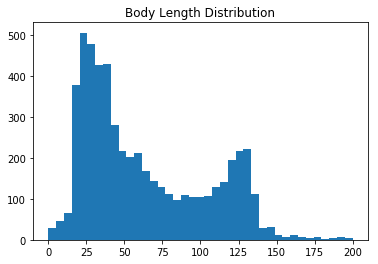#### Buidling 2 Histogram to look at the full distrbution % of text that is punctuation for spam and ham¶

In :
# definining bins for hitogram using numpy
bins = np.linspace(0, 50, 40) # this is in %

# plot for spam distribution
pyplot.hist(data['punc%'], bins)

# title
pyplot.title('Punctuation % Distribution')

pyplot.show()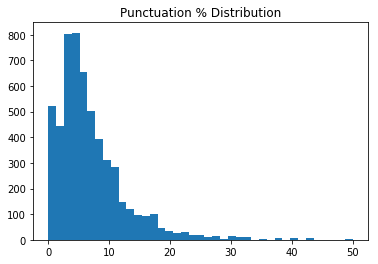### Box-Cox Power Transformations¶

Transfomation is a process that alters each data point in a certain column in a systematic way.

Base Form: $$y^x$$

X Base Form Transformation
-2 $$y ^ {-2}$$ $$\frac{1}{y^2}$$
-1 $$y ^ {-1}$$ $$\frac{1}{y}$$
-0.5 $$y ^ {\frac{-1}{2}}$$ $$\frac{1}{\sqrt{y}}$$
0 $$y^{0}$$ $$log(y)$$
0.5 $$y ^ {\frac{1}{2}}$$ $$\sqrt{y}$$
1 $$y^{1}$$ $$y$$
2 $$y^{2}$$ $$y^2$$

Transformation Process

1. Determine what range of exponents to test
2. Apply each transformation to each value of your chosen feature
3. Use some criteria to determine which of the transformations yield the best distribution
In :
# creating the for loop to loop through some different transfomation and plot the data with that transfomation applied
for i in [1,2,3,4,5]:
pyplot.hist((data['punc%'])**(1/i), bins=40)
pyplot.title("Transformation: 1/{}".format(str(i)))
pyplot.show()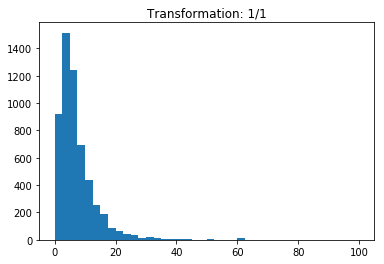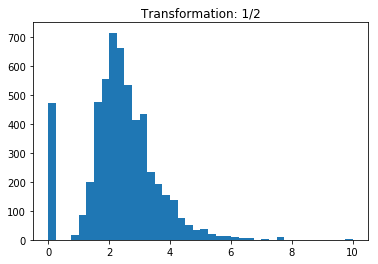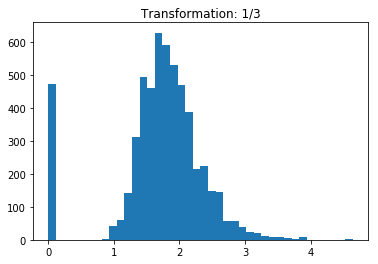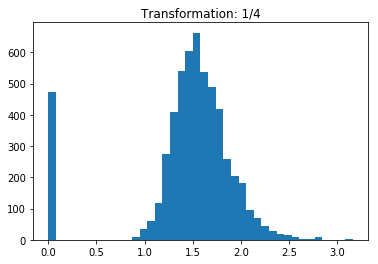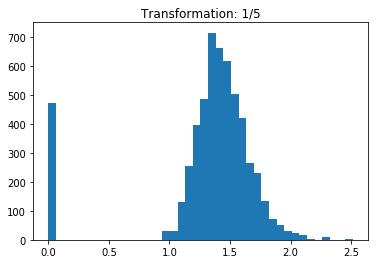Author: Amandeep Saluja